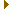# mightymandel v16

## GPU-based Mandelbrot set explorer

utility.c File Reference

### (v16)

Miscellaneous utility functions. More...

#include <math.h>
#include <stdio.h>
#include "utility.h"Include dependency graph for utility.c:

Go to the source code of this file.

## Functions

int min (int a, int b)
Find the minimum of two ints.
int max (int a, int b)
Find the maximum of two ints.
int ceil2n (int z)
Find the next power of two.
int pxbits (const mpfr_t radius, double height)
Find the precision needed to represent pixel coordinates in an image.
void pixel_coordinate (mpfr_t x, mpfr_t y, int width, int height, const mpfr_t centerx, const mpfr_t centery, const mpfr_t radius, double i, double j)
Find the coordinates corresponding to a pixel.

## Detailed Description

Miscellaneous utility functions.

Definition in file utility.c.

## Function Documentation

 int ceil2n ( int z )

Find the next power of two.

Parameters
 z A number.
Returns
The least power of two greater or equal to the input number.

Definition at line 39 of file utility.c.

 int max ( int a, int b )

Find the maximum of two ints.

Parameters
 a First number. b Second number.
Returns
The maximum of the two numbers.

Definition at line 31 of file utility.c.

Referenced by find_blobs0(), parse_command_line(), and vram_available().Here is the caller graph for this function:

 int min ( int a, int b )

Find the minimum of two ints.

Parameters
 a First number. b Second number.
Returns
The minimum of the two numbers.

Definition at line 22 of file utility.c.

Referenced by find_blobs0(), and parse_command_line().Here is the caller graph for this function:

 void pixel_coordinate ( mpfr_t x, mpfr_t y, int width, int height, const mpfr_t centerx, const mpfr_t centery, const mpfr_t radius, double i, double j )

Find the coordinates corresponding to a pixel.

Parameters
 x Output real coordinate. y Output imaginary coordinate. width The image width in pixels. height The image height in pixels. centerx The real coordinate of the view center. centery The imaginary coordinate of the view center. radius The radius of the view. i The pixel x coordinate (0 is left of the image). j The pixel y coordinate (0 is top of the image).

Definition at line 76 of file utility.c.

Referenced by find_ref(), fpxx_start(), interact_coord(), interact_translate(), and tiling_next().Here is the caller graph for this function:

 int pxbits ( const mpfr_t radius, double height )

Find the precision needed to represent pixel coordinates in an image.

Parameters
 radius The radius of the view. height The height of the view in pixels.
Returns
The precision for pixels in the view.

Definition at line 54 of file utility.c.

Referenced by interact_center(), interact_zoom_about(), and render_options_set_location().Here is the caller graph for this function: